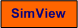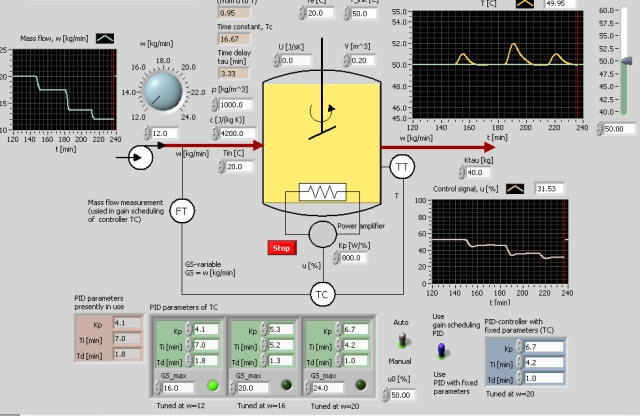# Gain Scheduling

Snapshot of the front panel of the simulator:• What is needed to run the the simulator? Read to get most recent information!
• Tips for using the simulator.
• The simulator: . The simulator runs immediately after the download by clicking Open in the download window. Alternatively, you can first save a copy of the exe-file on any directory (folder) on your PC and then run the exe-file, which starts the simulator.

## Description of the simulated system

A temperature control system of a heated stirred liquid tank with continuous throughflow may vary, is simulated, see the front panel of the simulator. It can be shown (see below) that the process dynamics (gain, time constant, time delay) largely depends on the mass flow through the tank, so the PID parameters should be be adjusted as functions of the mass flow to maintain satisfactory dynamics (as good stability) of the control system. In other words: Gain scheduling may be necessary.

#### About the process to be controlled

A process model can be derived from an energy balance (it is for simplicity assumed that the heat transfer through the walls is zero, i.e. U = 0, see the front panel of the simulator):

(1) d(crVT1)/dt = cw(Tin - T1) + P

where the power P [W] is given by

P = KP*u

where u [%] is the control signal.

T1 is the temperature of the liquid in the tank assuming homogenous conditions. In practice there is a time delay t in the response in the temperature sensor relative to an excitation in the supplied power P. Thus,

(2) T(t) = T1(t-tau)

We assume that the time delay is inversely proportional to the mass flow w:

(3) tau = Ktau/w

#### The nominal operating point

The nominal operating point is charactericed as follows:

• The tank temperature T is 50 degrees C,
• The inlet flow temperature Tin is 20 degrees C.

#### The controller

In the simulator the gain scheduling PID controller of the PID Control Toolset in LabVIEW is used. In this controller a piecewise constant interpolation is used between the different PID parameter sets. In each interval the PID parameter values are held constant at a conservative value, which implies that the stability is ensured during the interval.

## Aims

The aims of the tasks below are as follows:

• To demonstrate that the dynamics of a process may vary with process parameter value, here: the mass flow through the system.
• To calculate proper PID settings for various mass flows, to be used in a gain scheduled PID controller
• To observe the benefits of gain scheduling (i.e. an adaptive controller) compared to a controller with fixed parameter values.

## Motivation

Gain scheduling is an important practical way to implement an adaptive PID controller, which means that the controller is continuously adapted to the process it controls. Gain scheduling is available in many commercial PID controllers.

A typical kind of processes which may require gain scheduling (i.e. controller adaptivity) is processes with varying mass flow, as in the present simulator. Concrete examples of such processes are heat exchangers and continuous reactors being in a process line with varying production rate.

1. Varying process dynamics:
1. From the model equations (1), (2) and (3) above, derive the transfer function HP(s) from the control signal u [%] to the temperature T. This transfer function becomes of first order with time delay. Show that the transfer function has the following parameters:

(4) gain Ku = KP/(cw)

(5) time constant Tc = rV/w

(6) time delay tau = Ktau/w

Thus, the process dynamics varies with the mass flow w.

2. Run simulations to demonstrate that Ku, Tc and t depends on the mass flow w as found in Task a above. Qualitative resultes are sufficient (thus, you are not expected to calculate the values of the gain, time constant and time delay for different values of w). (Tips for the simulations: Set the controller in manual mode. Change the supplied power as a step from the value, P0 (found experimentally, although you may calculate it from the model), which keeps the process at the operating point, and observe the response in the tank temperature.

2. PID controller with fixed parameters:
1. Controller tuning: Find proper PID settings for w = 20 (using e.g. the Ziegler-Nichols' closed loop method). Alternatively you can use the parameter values shown on the front panel of the simulator.
2. The dynamic behaviour of the control system when the process dynamics varies: Reduce the mass flow w to its smallest value (12 kg/min). How does the process parameters K, Tc and tau vary (the values are calculated and shown on the front panel of the simulator)? Is the stability of the control system still satisfactory? Does it seem to be need to adjust the PID settings as a function of w?
3. Choosing mass flow for conservative controller tuning: By conservative controller tuning is meant that the control system is guaranteed to not have bad stability. Which value of the mass flow w should be chosen for conservative controller tuning? (You do not have to run simulations to give an answer..)

3. Gain scheduling (adaptive) PID controller:
1. Controller tuning: Tune the PID controller (using e.g. the Ziegler-Nichols' closed loop method) for the following three w values: 16, 20 and 24 kg/min. Alternatively you can use the PID settings shown on the the front panel of the simulator. The above mentioned w values are used as the gain scheduling parameter P, see the front panel of the simulator.
2. The dynamic behaviour of the control system when the process dynamics varies: Use a gain scheduling PID controller. First, set the mass flow w at its maximum value, 24 kg/min, and let the controller system stabilize for this w value. Then, reduce the w value to 12 kg/min. This reduction may be in the form of three stepwise changes as follows: From 24 to 20, from 20 to 16, from 16 to 12). Observe that both the process parameters and the controller parameters varies. Is the stability of the control system satisfactory for any mass flow? Is gain sheduling (adaptivity) beneficial compared to using a controller with fixed settings, cf. Taks 2 above?

[SimView] [TechTeach]

Updated 19. January 2008. Developed by Finn Haugen. E-mail: finn@techteach.no.